# Sampling & Survey #10 – Cluster SamplingSo today we discuss about Cluster Sampling. We will begin by looking at cluster sampling with equal probabilities. Firstly, a cluster is a grouping of the members of the population. Previously, we assumed that we can directly sample the elements of the population and these sampling units are the elements of the population. However, this sampling frame list of elements might not be easily obtained due to cost constraints or limitations such as access to information.
An example: If we want to study residents of Singapore (Population), the element is the person. However, we may not be able to contact them directly, and can only find information of the household (cluster).

• In cluster sampling, the clusters are also called primary sampling units while the elements are called secondary sampling units.
• In cluster sampling, the sampling units are the clusters and the elements observed are the secondary sampling units within the clusters. Previously, we have treated  our primary sampling units as secondary sampling units.

Why is there a need to do cluster sampling?
Firstly, constructing a sampling frame list of observations units may be difficult, expensive or impossible.
Secondly, the population may be widely distributed geographically or may occur in natural clusters and it may be more cost efficient to take a sampling of clusters rather than an SRS of individuals.

As before, we have a lot of notations to follow first. And before that, I’ll illustrate a scenario here first. Suppose that our population consists of clusters of elements.
Cluster 1: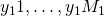SubtotalCluster 2: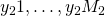SubtotalCluster 1: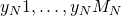Subtotalis the measurement for theelement in the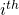cluster.
N = number of clusters in the population= number of elements in the cluster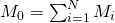is the number of elements in the populationis the average cluster size for the populationis the subtotal for theclusteris the population totalis the population variance of the cluster totals.is the population variance per elementis the population variance within cluster i

Finally, we are done with the notations and can finally ask ourselves how do we draw a cluster sample? We will study two approaches here, one-stage cluster sampling and two-stage cluster sampling. We will do the former now, and the latter in the next post.

One-Stage cluster sampling means that every element within a sampled cluster is included in the sample. Here, the populationelements are divided in N clusters of size.

TBC…

Sampling & Survey #1 – Introduction
Sampling & Survey #2 – Simple Probability Samples
Sampling & Survey #3 – Simple Random Sampling
Sampling & Survey #4 – Qualities of estimator in SRS
Sampling & Survey #5 – Sampling weight, Confidence Interval and sample size in SRS
Sampling & Survey #6 – Systematic Sampling
Sampling & Survey #7 – Stratified Sampling
Sampling & Survey # 8 – Ratio Estimation
Sampling & Survey # 9 – Regression Estimation
Sampling & Survey #10 – Cluster Sampling
Sampling & Survey #11 – Two – Stage Cluster Sampling
Sampling & Survey #12 – Sampling with unequal probabilities (Part 1)
Sampling & Survey #13 – Sampling with unequal probabilities (Part 2)
Sampling & Survey #14 – Nonresponse

Not readable? Change text.